# Numerical Simulation of Flow Around Two Side-by-Side Circular Cylinders at High Reynolds Number

Numerical Simulation of Flow Around Two Side-by-Side Circular Cylinders at High Reynolds Number

Hamidreza Rahimi Xiaonan TangYaser Esmaeeli Ming Li Alireza Pourbakhtiar

Xi’an Jiaotong-Liverpool University, Suzhou 215123, China

University of Liverpool, Liverpool, L69 3BX, UK

Corresponding Author Email:
Xiao.Tang@xjtlu.edu.cn
Page:
77-91
|
DOI:
https://doi.org/10.18280/ijht.380109
3 April 2019
|
Accepted:
26 December 2019
|
Published:
31 March 2020
| Citation

OPEN ACCESS

Abstract:

The fluid flow around a cylinder is one of the classic issues in fluid mechanics because of its various applications. Cylindrical structures, both single and in the group patterns, are present in the design of cooling systems of nuclear power plants, hydro-structures, heat exchangers, chimneys, high buildings, power lines, cables and networks in air and water. In many engineering applications, Karman's vortex shedding produces flow-induced vibrations. To comprehend the fluid structures surrounding the cylinders, it is vital to understand some fundamental issues such as boundary layers, flow separation, free shear layer, sequence and dynamics of vortices. According to the limited studies conducted for the vertical arrangement of two cylinders in a flow with the high Reynolds number, there is no evidence of oblique flow or bi-stable pattern except in the case where cylinders are close to each other. In this paper, the impact of the vertical arrangement of two cylinders on flow with high Reynolds number has been investigated via numerical modelling. The results indicated that the flow pattern is irregular and unstable for denser arrangements while the propagation of vortices does not have any tendency in different spacing.

Keywords:

circular cylinder, supercritical Reynolds number, drag coefficient, lift coefficient, side-by-side arrangement

1. Introduction

Since the fluid flow around a cylinder widely exits and has many different applications, its study has drawn great attention to many researchers. There are many studies on the flow around an isolated single circular cylinder, such as papers [1-7] and monographs [8-10]. Cylindrical structures can be found in either single or group patterns, e.g. in the designs of cooling systems of nuclear power plants, hydro-structures, heat exchangers, chimneys, high buildings, power lines, cables and networks in air and water. In many engineering applications Karman vortex shedding is sensible for problems with flow-induced vibration and noise. A comprehensive study has been done on the fluid dynamics for the flow around a circular cylinder, including fundamental topics such as the boundary layer, separation, the free shear layer, the wake and the dynamics of vortices. Therefore, fluid dynamics of the multiple immersed cylinders in a uniform vertical flow has been studied by many researchers. One of the first experimental studies on two cylinders in a longitudinal and vertical arrangement was conducted by Biermann and Herrnstein . Studies on behaviour of the flow in vicinity of the cylinder group in the vertical flow have been reviewed by researchers [12-15], and a recent major review in this regard was given by Sumner .

Furthermore, many experimental studies have been carried out on cylinders with the vertical arrangement in a steady horizontal flow, which varies from low Reynolds number (Re=50) to high Reynolds number (Re=6.5x105). Some experimental studies have been done on three cylinders with the vertical arrangement, for example the studies [17-25]. Study on a large number of cylinders in a single-row vertical arrangement has also been undertaken by researchers [26-30]. The results of such studies indicated that in such cases, the flow pattern is disordered, which is due to the mixing and the deviation of the flow through empty space between the cylinders, especially where T / D <2, where T is the distance between the centers of two cylinders and D is the diameter of the cylinders. Flow through empty space between the cylinders may frequently alternate, resulting in intermittent mixing of different jets passing through the cylinders.  This feature has led to the flow pattern called “Metastable" . In numerical analysis, studies have also been conducted on the flow around two cylinders in a vertical arrangement, which is mainly in lower Reynolds numbers , including the studies conducted by Chang and Song  for Re=100,  for Re=200,  for Re=100, 200, and  for Re=750.

Cylinders in different shapes and geometries are widely used in different engineering and modeling topics such as cooling system, pin-fins, vegetation. The flow around cylinders has continuously been investigated by numerous researchers to enhance the understanding of hydraulic properties of cylinders. Many researchers consider the relationship between the performance and geometry based on traditional circular dowels in common shape, such as of variable shapes [35, 36], different arrangements [37, 38] and geometric parameters [39-41] of cylinders. Additionally, several perforated features such as pin-fins are conceived to achieve favorable hydraulic and thermal performance. Results of relevant research indicate that there is a strong correlation between morphological feature and performance, such as the numbers or shapes of holes [42-44]. However, it seems difficult to make further improvement on performance based on geometrical modification or combination of multiple shapes. Therefore, this study seems to be essential for further studies in different aspects of engineering topics.

Theoretically, heat transfer efficiency can be improved by increasing the contact area and flow state using the lattice structure, as demonstrated through simulation and experiment [45, 46]. Therefore, some studies were performed continually to investigate the properties of lattice structure materials used in the different types of systems. Wei et al.  proposed a C/SiC lattice sandwich panel in the shape of pyramidal core and studied the equivalent thermal conductivity with a varied heat source. The thorough experiment of compacted heat exchange by using pyramidal latticed structure was conducted by Son et al.  to explore the influence of the geometrical parameter on hydraulic and thermal performance under the condition of laminar flow. Besides, several morphologically modified structures such as X-type lattice [49, 50] were proposed to enhance the thermal performance and to improve heat dissipation efficiency. The angle distribution of ligament in X-type lattice structure was demonstrated to be correlative with hydraulic and thermal performance.

Fewer studies are carried out about two or more cylinders located adjacently to each other. The flow field around the composition of multiple cylinders involves the complex interaction among shear layers, vortices, sequences, and streams of Karman's vortices. The most basic arrangement for combining multiple cylinders with the same diameter D is shown in Figure 1. For "unlimited" cylinders where the ratio of each cylinder, AR=H / D (H=height or cylinder length), is large enough to allow a two-dimensional flow around the cylinder, the main variables that control the flow behavior are: (1) the distance between the cylinders, (2) the orientation of cylinders in the xy plane relative to the flow direction, and (3) the Reynolds number (Re). Most studies have defined the Reynolds number by the uniform flow velocity U and the cylinder diameter D as Re=UD/v, where v is the kinematic viscosity of fluid. For a "limited" cylinder that is installed perpendicularly to a ground plane, the additional variables that control the behaviour of the fluid are (4) the dimensional ratio of the cylinder, and (5) the characteristics of the boundary layer of the ground plane.

The two ideal forms of cylinder orientation adjacent to each other are the longitudinal and transversal arrangement, as presented in Figure 1 (a) and (b), where the geometry with the spacing between centers of the cylinders is specified by longitudinally (L) and transversally (T). In such a case, the spacing between the cylinders is commonly defined as non-dimensional to longitudinal and transversal dimensions: length ratio (L/D) or transverse ratio (T/D).

## 9.1.png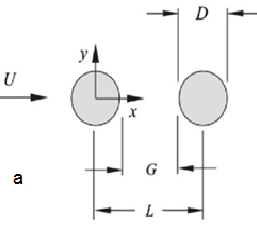## 9.2.png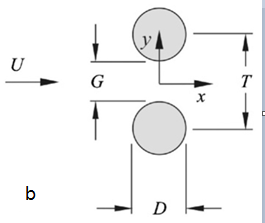Figure 1. Two cylinders with the same diameter in the transverse flow: (a) longitudinal arrangement and (b) vertical arrangement 

Numerous numerical and experimental studies have been done to understand the flow structure around two cylinders with the vertical arrangement in the low Reynolds number. Various patterns for the flow behaviour behind the cylinders have been identified and categorised. One of the most prominent characteristics of flow is the pattern of the oblique flow that occurs in the moderate ratios of T/D (1.1-1.2 < T / D < 2-2.2) and sometimes in the small ratios of T/D. Moreover, the unsteady biased behaviour of oblique flow is also oscillating between the cylinders.

One of the limitations in these kinds of numerical studies is only to study different coefficients and parameters in the same time, along with to understand what distance can have no effect on side dowel. According to the limited studies conducted for the vertical arrangement of two cylinders in a flow with the high Reynolds number, there is no evidence of oblique flow or bi-stable pattern except in the case of very small T/D arrangements (T/D= 1.1-1.2). The objective of this paper is to investigate the flow behaviors in various other arrangements. More specifically, this study is to model the fluid flow around two cylinders with a vertical arrangement in the Reynolds number 1x106 to investigate how the distance between cylinders affects the flow characteristics.

In the subsequent context of the paper, research methodology is first introduced in section 2, followed by a detailed numerical modelling in Section 3. The modelling results for single and two cylinders are represented in Sections 4 and 5, and conclusions are drawn in Section 6.

2. Research Methodology

2.1 Vertical arrangement (in y direction)

When two cylinders are located in the horizontal flow direction with a transverse arrangement, the cylindrical sequence interacts with each other on both sides of the empty space. If two cylinders are located in close vicinity, they would possibly act like an air-block body with an added "base bleed" effect . If the cylinders are located apart enough, they act as two separate air-block bodies, although they may be synchronised between nearby streets. When the cylinders are placed between the two spaces, there exists the complex interference between the sequence and the vortex street, resulting in an asymmetric and distorted flow pattern that is unstable and bi-stable. The transverse layout of cylinders is placed in the range of proximity interference defined by Wood .

In this case, the behaviour of the fluid is mainly a function of the ratio of centre-to-centre distance to the diameter of the cylinders (T/D) (in some studies, using the gap space to the diameter of the cylinders (G/D). It should also be mentioned that the Reynolds number affects flow characteristics, but its influence is less than the effect of longitudinal arrangement of the cylinders.

2.2 Evaluation of fluid flow in supercritical flow condition

Despite numerous studies on the flow behavior of fluid around cylinders, the measured data for the studies in low and high Reynolds numbers are very limited. In the supercritical flow that has a high Reynolds number, the effects of the wake interference are less for the cylinder arrangements .

According to the studies conducted by Sun et al. , the pattern of flow around cylinders with vertical arrangement in the high Reynolds number is symmetric in different states, which is in contrast to the flow pattern in the low Reynolds number, and only in the case of very small T/D (= 1.1-1.2) has the asymmetric flow pattern been observed.

Furthermore, no observation has done on no unsteady behaviour and bi-stable form of flow in the high Reynolds number. Gu  has categorised the supercritical interference in two modes: (a) the shear layer interference and (b) the interference of sequences or neighbouring effects (Figure 2).

## 9.2a.png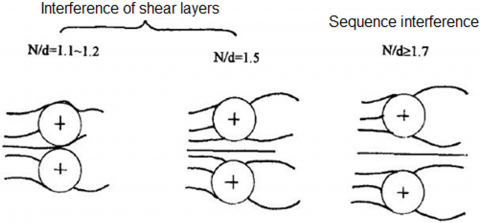Figure 2. Classification of the flow around two cylinders with vertical arrangement in the high Reynolds number (Re=4.25×105), where N is the distance between the center of two cylinders and d is the diameter of the cylinders

Only a limited number of studies have been carried out with focusing on the behaviour of fluid flow around cylinders that have a transverse layout in high Reynolds number, along with the inaccuracy of the values of drag coefficients and their variations with the distance between the cylinders. So further investigations are needed in this regard.

3. Numerical Modelling

Solving differential equations of fluid motion numerically is called Computational Fluid Dynamics, commonly known as CFD, which deals with the numerical solutions of fluid flow parameters. The physical laws governing the flow of fluid are brought up by partial differential equations such as the continuity and the Navier-Stokes momentum or any other additional equations. Numerical solvers solve these equations with acceptable precision using complex computation methods as a program or code, in which the algorithm is written and runs on a computer.

3.1 Governing equations

The governing equations, including the continuity and momentum equations, are written for the three-dimensional, incompressible, unsteady flow:

$\frac{\partial \tilde{u}_{i}}{\partial x_{i}}=0$   (1)

$\frac{\partial \tilde{u}_{i}}{\partial t}+\tilde{u}_{j} \frac{\partial \tilde{u}_{i}}{\partial x_{j}}=-\frac{1}{\rho} \frac{\partial \tilde{p}}{\partial x_{i}}+\frac{\partial}{\partial x_{j}}\left(2 v \tilde{S}_{i j}\right)$    (2)

where, the subscripts i and j are the Cartesian coordinates, $\tilde{u}_{i}$ represents the components of instantaneous velocity, $\tilde{p}$ is the instantaneous pressure, $\rho$ is the fluid density, $v$ is the kinematic viscosity of fluid, $t$ and $x_{i}$ represent the time and coordinate components $(i=1,2,3),$ respectively. For the incompressible fluid, the density is assumed to be constant throughout the computation $[56-62] .$ The strain tensor is defined as follows:

$\tilde{S}_{i j}=\frac{1}{2}\left(\frac{\partial \tilde{u}_{i}}{\partial \mathrm{x}_{\mathrm{j}}}+\frac{\partial \tilde{u}_{j}}{\partial \mathrm{x}_{\mathrm{i}}}\right)$   (3)

3.2 Validation of the basic model

Considering that this study aims to understand the flow field around two cylinders in a vertical arrangement with a high Reynolds number Re =1X106 , the flow around the single cylinder in the high Reynolds number was first modelled in ANSYS-Fluent commercial code, and the results are  then compared with experimental and numerical studies to verify the validity and accuracy of the discretisation of CFD turbulence models with the assigning boundary conditions and other important parameters of the flow. This process includes (1) modelling of the flow around the single cylinder with Re=1x106 and (2) geometric modeling and dimensions of the computational domain.

In the modelling of two-dimensional flow, to minimise the effect of the boundaries, the width dimensions of the rectangular computation domain are considered to be large enough so that they were set to be 24D X28D , where D is the diameter of the cylinder and equal to 2 meters. The free flow is entered from the left side of the domain at a velocity of U=7.3 m/s, and the coordinates of the centre of the cylinder in the field are set to at P(8D, 12D); the position of origin is indicated clearly in Figure 3, which is the computational domain for the single cylinder in the study.

In Table 1, the values of the Strouhal coefficient ($S t=\frac{f L}{U}$, where f is the frequency of vortex shedding, L is the characteristics length and U is the flow velocity) are presented along with the mean drag coefficient (CD), and maximum mean lift coefficient (CL) in the turbulent flow around the single cylinder for Re=1x106, in six meshes.

Table 1. The mean drag coefficient, the mean maximum lift coefficient and Strouhal number around the single cylinder in the flow with Re=1x106

 St CL CD Number of cells Mesh 0.33 0.85 0.6 42,000 A 0.33 0.9 0.58 78,000 B 0.33 0.75 0.55 98,000 C 0.33 0.19 0.36 170,000 D 0.33 0.2 0.36 230,000 E 0.32 0.22 0.36 262,000 F

## 9.3.png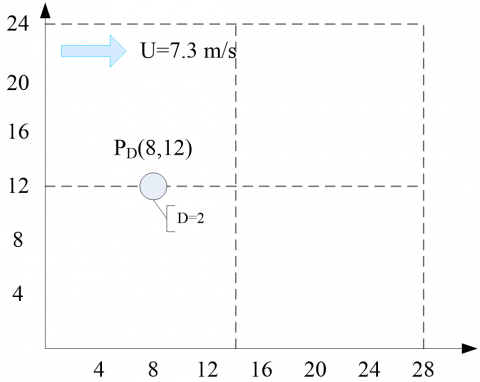Figure 3. Computational domain for a single cylinder

In order to obtain the independent results free from the effect of cell sizes, also described as mesh sensitivity analysis, six meshes with different element sizes have been generated in ANSYS-ICEM CFD. Mesh sensitivity parameters are selected as drag coefficient, lift coefficient and Strouhal number. The results of mesh sensitivity analysis are shown in Table 1, which shows that the increasing number of grid cells more than 170,000 does not change the results very much. Thus, the generated mesh with 170,000 cells was used in the modelling for the numerical analysis of flow characteristics.

3.3 Meshing

To obtain the independent results from cell sizes, six meshes with different sizes have been generated in ANSYS-ICEM CFD. In this study, the results of mesh sensitivity from cell sizes have been proven, showing that changing the sizes of mesh to certain degrees does not change the simulation results in terms of CD, CL and St., as shown in Table 1.

Table 1 shows that the finer dimension of the grid has little impact on the drag and lift coefficients from mesh D to F, indicating that the results are independent of the mesh sizes. Therefore, due to the timing of computation, the mesh D was selected as the optimal mesh, whose characteristics are presented in Figure 4.

## 9.4a.jpg## 9.4b.jpg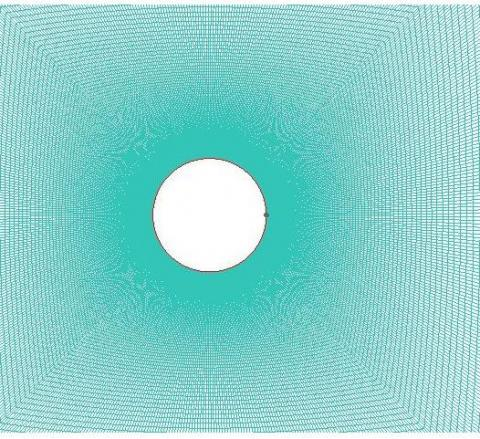Figure 4. Meshing details of computation domain using an optimal mesh D

The number of nodes used on the grid edges is also presented in Figure 4. The O-grid block was used around the cylinder with 200 points assigned to its edge perpendicular to the cylinder.

Since the velocity gradients are very large in the direction perpendicular to the wall around the cylinder, the boundary layer mesh was used close to the wall, and the size of the first cell at the boundary of the cylinder wall was assigned to be 0.00014 m. After completing the meshing process, the final mesh was transferred to the ANSYS-Fluent solver, and then the boundary conditions and characteristics of the problem and other parameters were defined.

3.4 Boundary conditions

3.4.1 Conditions of inlet and outlet boundaries

The inlet and outlet boundaries are sections where the fluid enters and leaves the computational domain, respectively. These boundaries are usually set as the conditions at a specified velocity or with a specific pressure. Because of the pairing of the pressure and velocity in the momentum equations, only one of the two conditions of pressure or velocity is defined. For the inlet boundary condition, the velocity value is determined along the x coordinate with the value of U=7.3 m/s, and for the outlet boundary condition, the static pressure is determined through the outlet, which is set to be the same atmospheric pressure, i.e. zero in relative pressure. For the parameters of turbulence intensity in the inlet and outlet, the following relationships are used :

$I=0.16(\mathrm{Re})^{1 / 8}$    (4)

$\ell=0.07 \mathrm{D}$   (5)

where, I represents the turbulent intensity defined as follows:

$I=\acute{u}_{i} / u_{i}$   (6)

where, $\acute{u}_{i}$ is the root mean square of the turbulent velocity fluctuations and ui is the mean velocity. The length of the turbulence scale (l) in Eq. (5) indicates the size of the large vortices in the turbulent flow, and D is the diameter of the cylinders. The values of I and l are 0.9 and 0.14, respectively.

3.4.2 Boundary conditions of the wall

For cylinder arrangement, a wall boundary condition is used. Since the fluid does not cross the wall, the vertical component of the velocity relative to the wall is zero. In addition, due to the no-slip wall condition, the tangential component of the velocity is also zero. In the upper and lower side boundaries, the vertical component of the velocity is considered zero, and the tangential component of the velocity remains unchanged. So, the symmetry boundary condition is used (i.e. no-shear wall).

3.4.3 Models in the ANSYS-Fluent solver

In the present analysis, an incompressible fluid of air with a density $\rho=1.225\left(k g / m^{3}\right)$ and dynamic viscosity $\mu=1.78 \times 10^{-5} k g /(m . s)$ was used. The SST (Shear Stress Transport) model was used to model turbulence. The SIMPLE algorithm was used to solve the governing equations, and all equations were performed with second order upwind discretization. For convergence of solutions, the model was first solved in the steady state, then solved in the unsteady state and time-variant with the time step of 0.05s and the number of time steps of 600 to 700.

4. Results for Single Cylinder

After the simulation is completed by the ANSYS-Fluent solver, the resulted data are processed in the CFD-Post component.

The flow pattern around the single cylinder in the flow for Re=1x106 and turbulent eddy viscosity contours are illustrated in Figures 5 and 6, respectively. The phenomenon of vortex shedding and vortex street behind the cylinder is well illustrated in these figures.

## 9.5.png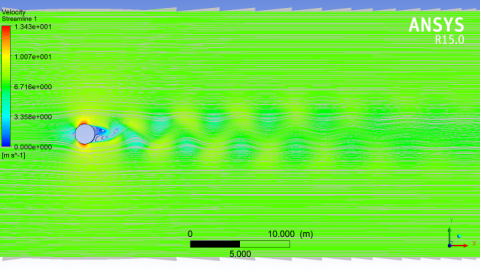Figure 5. Flow streamlines around the single cylinder with Re=1x106

## 9.6.png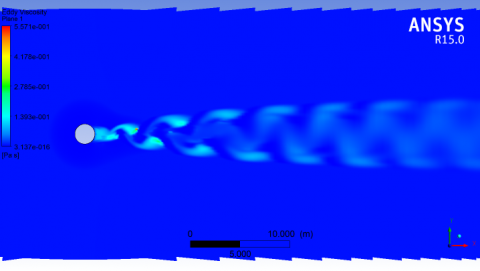Figure 6. Turbulent eddy viscosity contours of a single cylinder with Re=1x106

## 9.7.png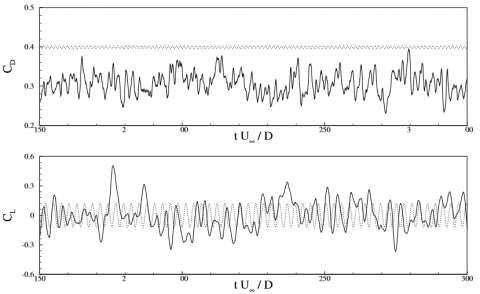Figure 7. Dash-line is the numerical results for RANS turbulence models, and continuous-line is the numerical results from LES (Large Eddy Simulation) 

Furthermore, the study  shows the variations of the drag and lift coefficients with time, as seen in Figure 7. For comparison purpose, Figure 8 illustrates an overall variation of CD with the Reynolds number in the single cylinder for experimental studies by various researchers . The mean value of CD  for Re=1x106 is obtained between 0.24 and 0.4.

This study focuses on lift and drag coefficients in the vertical arrangement of cylinders. Thus, in the following context, diagrams and values of the lift and drag coefficients are compared and validated for experimental and numerical studies that are performed on flows with the single cylinder in Re=1x106.

For the convenience of comparison, the results of this study and the previous numerical and experimental studies are presented in Table 2, in which the mean drag coefficient is CDm, mean lift coefficient is CLm and the Strouhal number is St.

The values of the mean drag coefficient (CD) and average lift coefficient (CL) in the present study were obtained to be 0.36 and 0, respectively, and the mean of maximum values for the lift coefficient was 0.19. In this respect, the results of our study correspond well to the results of the previous studies.

## 9.8.png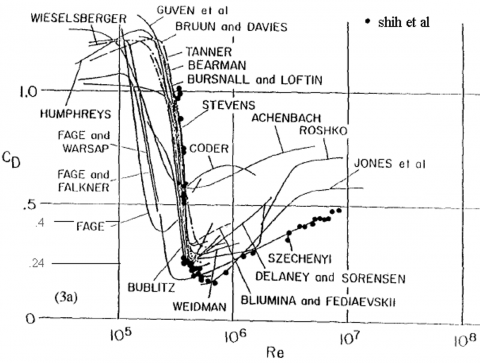Figure 8. Medium drag coefficient for the single cylinder relative to Reynolds number in various laboratory studies 

The Strouhal number was between 0.18 and 0.5 for the single cylinder with Re=1x106 in laboratory studies , and in the numerical study by , the Strouhal number was 0.31 for RANS turbulence models and 0.35 for the LES analysis. Given the Strouhal number of 0.33 in Table 1, our modelling results also correspond well to the previous experimental and numerical studies.

Table 2. Comparison between the results of current study and of previous numerical and experimental studies

 Studies CDm CLm St The present study 0.36 0 0.33 LES simulation  0.31 0 0.35 RANS simulation  0.40 0 0.31 Experimental studies  0.24-0.40 - - Experimental studies  0.17-0.40 - 0.18-0.50
5. Numerical Results of Two Cylinders

This study intends to model the fluid flow around two cylinders with a vertical arrangement in the Reynolds number 1x106 to study the effect of the distance between cylinders on the drag coefficients and the Strouhal number. The computation domain is shown in Figure 9, where the diameter of the cylinders was similar to the single cylinder (2 meters) performed in the modelling of Section 4, and the flow characteristics and parameters required for modelling were also similar. Thus, the flow velocity was considered to be 7.3 m/s. After a comprehensive review about the effect of the distance between cylinders on the drag coefficients in the high Reynolds number condition, T/D=1.1, 1.3, 1.5, 2.5 and 5 were selected to reflect a reasonable range of T/D values in the study. The meshing of the model was similar to the single cylinder model where the O-grid block was used in the vicinity of the cylinders. The SST turbulence model was used in the simulation. For convergence of solutions, the modelling was first carried out in a steady state condition and then done in an unsteady and time-variant mode with the time step size and the number of time steps being 0.05 and 600, respectively.

The results are presented in the form of the variations of both drag and lift coefficients with time, along with streamlines that present the flow pattern around the cylinders. To distinguish the results, number 1 was used to denote the values for the lower cylinder and number 2 to denote the values for the upper cylinder. Figures 10-12 show the changes of drag coefficient and lift coefficient with the time, and the streamline for each state, respectively.

## 9.9.pngFigure 9. Study domain used to model two cylinders in a vertical arrangement

5.1 The results for T/D=1.1

Figures 10 and 11 show that the values of both the drag and lift coefficients for T/D= 1.1 arrangement change irregularly in the first 30 seconds and do not follow any pattern. This disorder and incompliance with a certain period are also evident in the vortex shedding pattern, as illustrated in Figure 12. Figure 11 also shows a high value of the lift coefficient for the two cylinders at first a few seconds, which indicates the existence of a low-pressure and a suction area between the cylinders at early time.

## 9.10a.png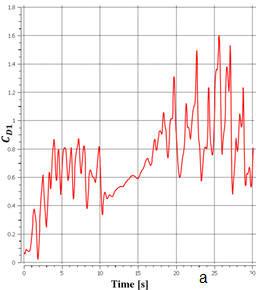## 9.10b.pngFigure 10. The drag coefficients for T/D=1.1: (a) cylinder 1 and (b) cylinder 2

## 9.11a.png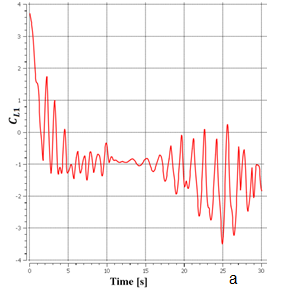## 9.11b.png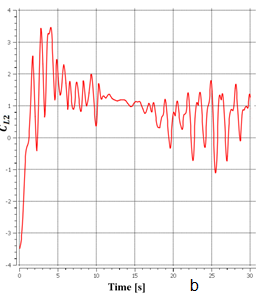Figure 11. The lift coefficients for T/D=1.1: (a) cylinder 1 and (b) cylinder 2

## 9.12.png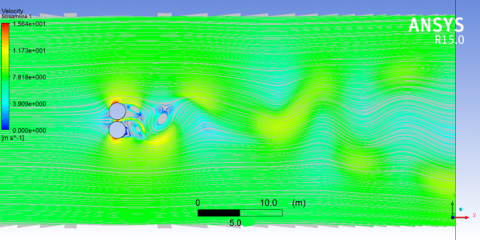Figure 12. Streamlines for T/D=1.1

5.2 The results for T/D=1.3

According to the results of Figures 13-15, the drag coefficient, the lift coefficient and the flow pattern for T/D=1.3 all change with time without following any pattern, similarly to those in T/D=1.1.

## 9.13a.png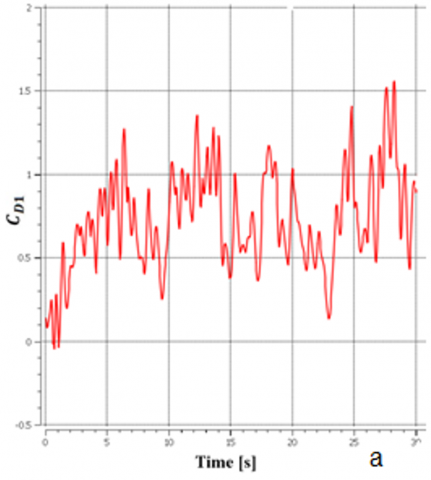## 9.13b.png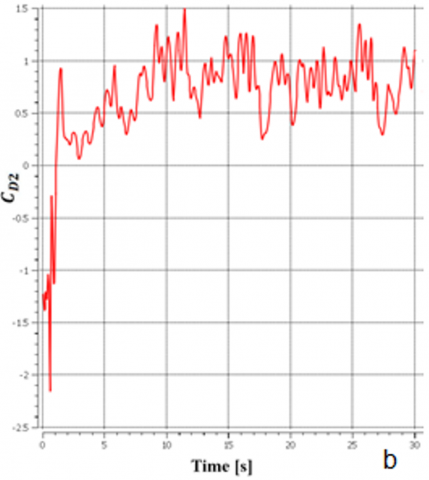Figure 13. The drag coefficients for T/D=1.3: (a) cylinder 1 and (b) cylinder 2

## 9.14a.png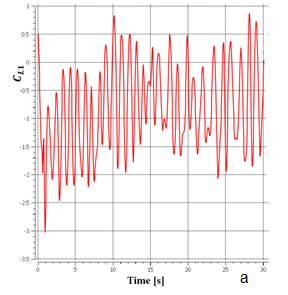## 9.14b.png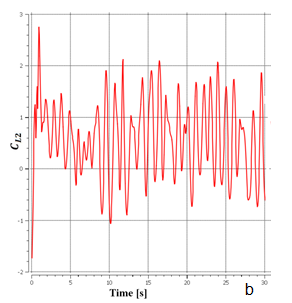Figure 14. The lift coefficients for T/D=1.3: (a) cylinder 1 and (b) cylinder 2

## 9.15.png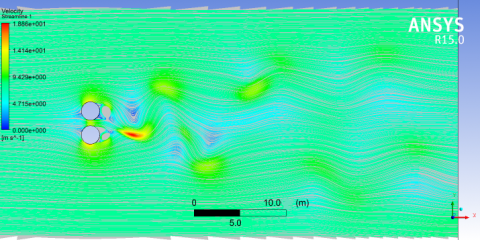Figure 15. Streamlines for T/D=1.3

5.3 The results for T/D=1.5

The drag coefficients of two cylinders are the same for T/D= 1.5 and have almost the same mean drag coefficient of 0.8 (Figure 16). Figure 17 indicates a similar oscillation of the lift coefficients for the two cylinders in T/D= 1.5 and that the mean value of the lift coefficient for each cylinder is approximately 0.3, which indicates the repulsive force exists between the two cylinders. According to Figure 18, the formation and shedding of vortices for two cylinders are inconsistent.

In short, the patterns of flow and oscillation of the drag and lift coefficients for the two cylinders are similar in T/D= 1.5, and the values of the mean drag and mean lift coefficients for each cylinder are almost the same and equal to 0.68 and 0.2, respectively. However, the formation and shedding of vortices in two cylinders are not in the same phase.

## 9.16a.png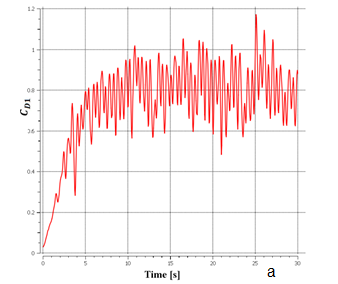## 9.16b.png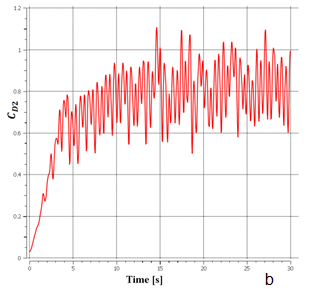Figure 16. The drag coefficients for T/D=1.5: (a) cylinder 1 and (b) cylinder 2

## 9.17a.png## 9.17b.png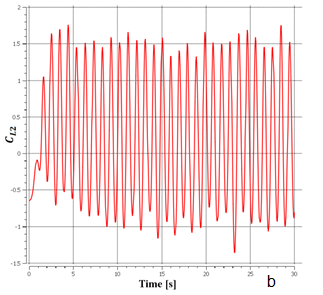Figure 17. The lift coefficients for T/D=1.5: (a) cylinder 1 and (b) cylinder 2

## 9.18.png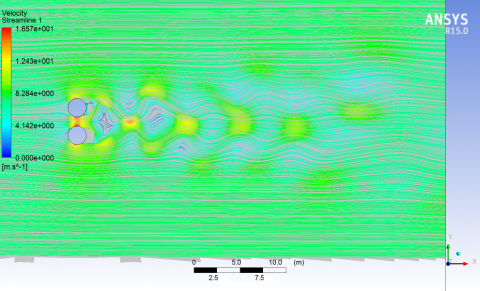Figure 18. Streamlines for T/D=1.5

5.4 The results for T/D=2.5

As shown in Figures 19-21, the patterns of flow and the oscillation of the drag and lift coefficients for the two cylinders are similar for T/D= 2.5 arrangement, and the values of the mean drag and mean lift coefficients for each cylinder are nearly the same and equal to 55.0 and 125.0, respectively. However, the formation and shedding of vortices in two cylinders are not in the same phase.

## 9.19a.png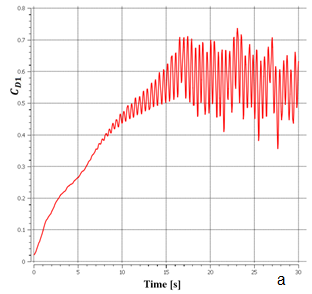## 9.19b.png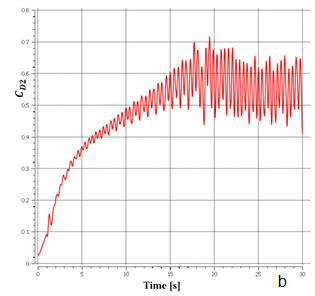Figure 19. The drag coefficients for T/D= 2.5: (a) cylinder 1 and (b) cylinder 2

## 9.20a.png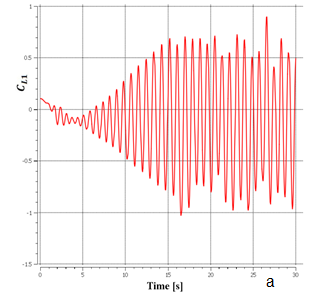## 9.20b.png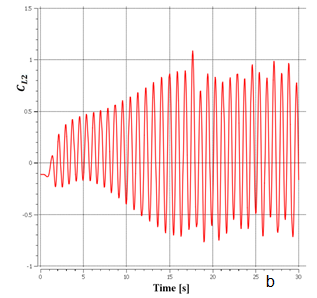Figure 20. The lift coefficients for T/D= 2.5: (a) cylinder 1 and (b) cylinder 2

## 9.21.pngFigure 21. Streamlines for T/D= 2.5

5.5 The results for T/D=5

As demonstrated in Figures 22-24, the patterns of flow and oscillation of the drag and lift coefficients for the two cylinders are similar or T/D= 5, and the values of the mean drag and mean lift coefficients for each cylinder are the same and equal to approximately 38.0 and 0.0 respectively. The formation and shedding of vortices in two cylinders are not in the same phase. In contrast, the obtained drag and lift coefficients are rather irregular and unstable for T/D=1.1 and 1.3.

To demonstrate how the mean drag and mean lift coefficients for two cylinders change with different T/D values, CD (averaged) and CL (averaged) against T/D are given respectively in Figs. 25 and 26, where the subscript m denotes the averaged value. Meanwhile, the values of the Strouhal number obtained from the diagrams of lift coefficients for different T/Ds are given in Table 3, which also includes the ratio of same-phased vortex shedding to time (same-phased and non-phased) for comparison.

Figures 25 and 26 show that both the mean drag and mean lift coefficients generally decrease as increasing T/D, especially with CLm declining rapidly in small T/Ds values up to 1.7. They also reveal that the mean values of the drag coefficient and the lift coefficient of cylinder 1 are higher than the corresponding values of cylinder 2 when T/D is small (less than 1.3), but there is almost no difference between the two cylinders for T/D >1. As the spacing between the two cylinders increases, the mean drag coefficient decreases and eventually approaches that value for the single cylinder if T/D increases to a value of approximate 4. The mean lift coefficient decreases with increasing spacing between the cylinders and gradually approaches the value for the single cylinder at a similar value of T/D. Table 3 shows the values of Strouhal number for both cylinders in different T/D arrangements, which indicates although Strouhal number was the same for both cylinders it changes in different T/Ds. The St. Number increases from 0.27 in T/D=1.5 to 0.33 at T/D=5.

## 9.22a.png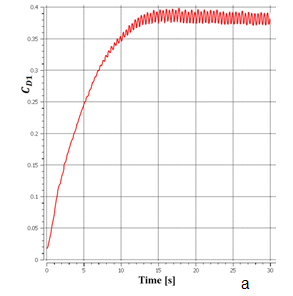## 9.22b.png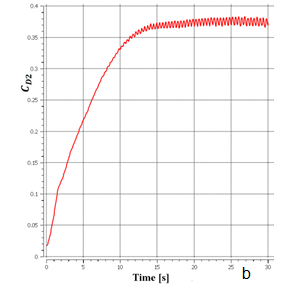Figure 22. The drag coefficients for T/D= 5: (a) cylinder 1 and (b) cylinder 2

## 9.23a.png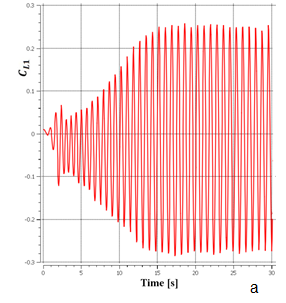## 9.23b.png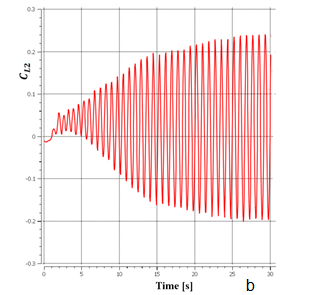Figure 23. The lift coefficients for T/D= 5: (a) cylinder 1 and (b) cylinder 2

## 9.24.png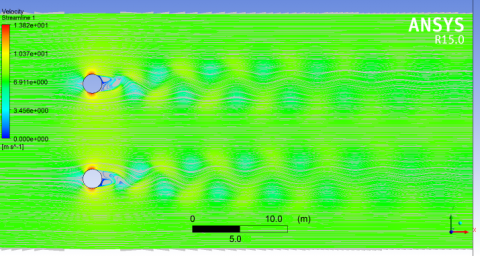Figure 24. Streamlines for T/D=5

## 9.25.png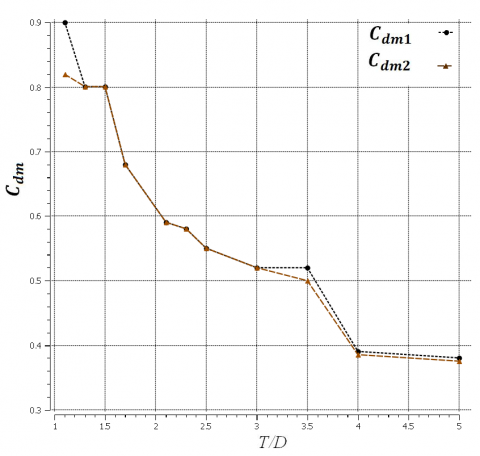Figure 25. Mean drag coefficients against T/D for two cylinders in the vertical arrangement for Re= 1x106

## 9.26.png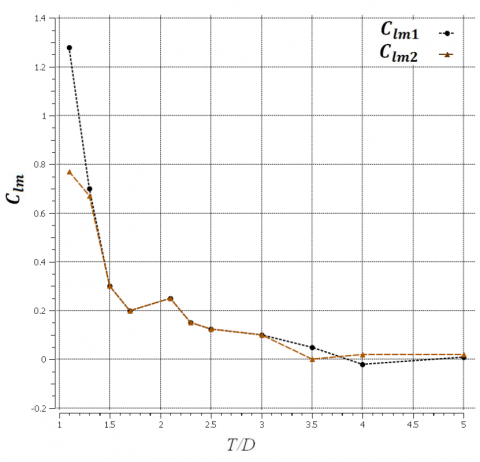Figure 26. Mean lift coefficients against T/D for two cylinders in the vertical arrangement for Re= 1x106

Table 3. Strouhal number valuesfor different T/D arrangements

 Phase condition St2 St1 T/D ----- ----- ----- 1.1 ----- ----- ----- 1.3 Non-phased 0.27 0.27 1.5 Non-phased 0.3 0.3 1.7 Same-phased 0.33 0.33 2.1 Non-phased 0.33 0.33 2.3 Non-phased 0.3 0.3 2.5 Non-phased 0.3 0.3 3 Same-phased 0.3 0.3 3.5 Same-phased 0.33 0.33 4 Non-phased 0.33 0.33 5
6. Conclusions

Based on the extensive studies for various T/Ds for two cylinders, the modelling results show (1) the main factors that are responsible for the fluid behaviour in the vicinity of the cylinders with the vertical arrangement in the high Reynolds number, and (2) the influence of the spacing between cylinders on the drag and lift coefficients. In the present paper, modelling has been undertaken for 11 different cases of T/D=1.1, 1.3, 1.5, 1.7, 2.1, 2.3, 2.5, 3, 3.5, 4, 5 with the Reynolds number of 1x106. The main results about CD and CL are presented in Figures 25 and 26.

The results indicated that the flow pattern is irregular and unstable for small T/D arrangements (T/D ≤ 1.3) when the propagation of vortices does not have any tendency. This result agrees with the results of studies conducted by Gu , and Gu et al. .

For higher T/D arrangements (T/D > 1.3), the flow pattern behind the cylinders is largely similar to that of the parallel vortex street pattern by  who placed cylinders in the condition of a low Reynolds number. Surely, this pattern has also been observed for the higher T/D arrangements (T/D > 2-2.2) in a high Reynolds number, and the shedding for both cylinders propagates at a similar frequency to the Karman’s vortex.

The two cylinders with a vertical arrangement act as separate air-block bodies. Thus, the effects of adjacent interference can cause different modes of same-phased or different-phased in the shedding of vortices, and parallel vortices streets are formed.

In this study for all the proposed arrangements, either the oblique flow or the bi-stable, behaviour of the flow was observed as they occur in the flow of low Reynolds number. This result agrees with the result of . The results of the drag and lift coefficients indicate that the values of both the average drag and lift coefficients for each cylinder are approximately the same for all our test cases except in T/D=1.1.

In the cases where T/D is very low, e.g. T/D=1.1, the values of the average lift and drag coefficients for cylinder 1 were greater than for cylinder 2. However, in other cases, as the spacing between cylinders increases, the values of the average lift and drag coefficients decrease and gradually approach the value of the drag coefficient for the single cylinder when the T/D is high (approximately at 4).

For higher T/D arrangements (T/D=4.5), the mean drag and the mean lift coefficients and the flow pattern for each cylinder are all similar to those for the single cylinder, indicating that the effects of shedding interactions are negligible, i.e. the flow around the cylinders is roughly independent.

References

 Morkovin, M. (1964). Flow around circular cylinders-a kaleidoscope of challenging fluid phenomena. In Proc. ASME Symp. on Fully Separated Flow, Philadelphia, pp. 102-118.

 Gerrard, J.H. (1978). The wakes of cylindrical bluff bodies at low Reynolds number. Philosophical Transactions of the Royal Society of London. Series A, Mathematical and Physical Sciences, 288(1354): 351-382. https://doi.org/10.1098/rsta.1978.0020

 Coutanceau, M., Defaye, J.R. (1991). Circular cylinder wake configurations: A flow visualization survey. Appl. Mech. Rev., 44(6): 255-305. https://doi.org/10.1115/1.3119504

 Williamson, C.H. (1996). Vortex dynamics in the cylinder wake. Annual Review of Fluid Mechanics, 28(1): 477-539. https://doi.org/10.1146/annurev.fl.28.010196.002401

 More, B.S., Chauhan, M.K., Dutta, S., Gandhi, B.K. (2017). Investigation of flow interference between two inline square cylinders with different spacing ratios in subcritical Reynolds number regime. In Fluid Mechanics and Fluid Power–Contemporary Research, 1293-1301. https://doi.org/10.1007/978-81-322-2743-4_123

 Silva-Ortega, M., Assi, G.R.D.S. (2017). Flow-induced vibration of a circular cylinder surrounded by two, four and eight wake-control cylinders. Experimental Thermal and Fluid Science, 85: 354-362. https://doi.org/10.1016/j.expthermflusci.2017.03.020

 Mysa, R.C., Law, Y.Z., Jaiman, R.K. (2017). Interaction dynamics of upstream vortex with vibrating tandem circular cylinder at subcritical Reynolds number. Journal of Fluids and Structures, 75: 27-44. https://doi.org/10.1016/j.jfluidstructs.2017.08.001

 Sumer, B.M., Christiansen, N., Fredsøe, J. (1997). The horseshoe vortex and vortex shedding around a vertical wall-mounted cylinder exposed to waves. Journal of Fluid Mechanics, 332: 41-70. https://doi.org/10.1017/S0022112096003898

 Zdravkovich, M.M. (1997). Flow Around Circular Cylinders Vol. 1: Fundamentals. Oxford University Press.

 Graham, J.M.R. (2003). Flow Around Circular Cylinders. Vol. 2: ApplicationsM. M. Zdravkovich, Oxford Science Publications (Oxford University Press): January 2003. Journal of Fluids and Structures, 18(1): 146-146. https://doi.org/10.1016/S0889-9746(03)00088-4

 Biermann, D., Herrnstein Jr, W.H. (1934). The interference between struts in various combinations. Langley Aeronautical Laboratory.

 Zdravkovich, M.M., Pridden, D.L. (1977). Interference between two circular cylinders; series of unexpected discontinuities. Journal of Wind Engineering and Industrial Aerodynamics, 2(3): 255-270. https://doi.org/10.1016/0167-6105(77)90026-5

 Nishimura, T. (1986). Flow across tube banks. In: Cheremisinoff, N.P. (Ed.): Encyclopedia of Fluid Mechanics-Flow Phenomena and Measurement, 1: 763-785.

 Chen, S.S. (1987). Flow-Induced Vibration of Circular Cylindrical Structures. Hemisphere Publishing Corporation, Washington, DC, USA.

 Ohya, Y.O., Okajima, A., Hayashi, M. (1989). Wake interference and vortex shedding. In: Cheremisinoff, N.P. (Ed.): Encyclopedia of Fluid Mechanics-Aerodynamics and Compressible Flow, 8: 322-389.

 Sumner, D. (2010). Two circular cylinders in cross-flow: A review. Journal of Fluids and Structures, 26(6): 849-899. https://doi.org/10.1016/j.jfluidstructs.2010.07.001

 Eastop, T.D. (1982). Air flow around three cylinders at various pitch-to-diameter ratios for both a longitudinal and a transverse arrangement. Trans. Inst. Chem. Eng., 60: 359-363.

 Kumada, M. (1984). Wake interference between three cylinders arranged side-by-side normal to a flow. Trans. JSME, 50: 1699-1707.

 Williamson, C.H.K. (1985). Evolution of a single wake behind a pair of bluff bodies. Journal of Fluid Mechanics, 159: 1-18. https://doi.org/10.1017/S002211208500307X

 Sumner, D., Wong, S.S.T., Price, S.J., Paidoussis, M.P. (1999). Fluid behaviour of side-by-side circular cylinders in steady cross-flow. Journal of Fluids and Structures, 13(3): 309-338. https://doi.org/10.1006/jfls.1999.0205

 Zhou, Y., So, R.M.C., Liu, M.H., Zhang, H.J. (2000). Complex turbulent wakes generated by two and three side-by-side cylinders. International Journal of Heat and Fluid Flow, 21(2): 125-133. https://doi.org/10.1016/S0142-727X(99)00077-6

 Zhang, H.J., Zhou, Y. (2001). Effect of unequal cylinder spacing on vortex streets behind three side-by-side cylinders. Physics of Fluids, 13(12): 3675-3686. https://doi.org/10.1063/1.1412245

 Wang, X.W., Zhang, H.J., Zhou, Y., Tu, J.Y. (2002). Flow visualization behind three cylinders of equal and unequal spacing. Journal of Flow Visualization and Image Processing, 9(2&3). https://doi.org/10.1615/JFlowVisImageProc.v9.i2-3.40

 Zhiwen, W.A.N.G., Zhou, Y., Li, H. (2002). Flow-visualization of a two side-by-side cylinder wake. Journal of Flow Visualization and Image Processing, 9(2&3). https://doi.org/10.1615/JFlowVisImageProc.v9.i2-3.30

 Zhou, Y. (2003). Vortical structures behind three side-by-side cylinders. Experiments in Fluids, 34(1): 68-76. https://doi.org/10.1007/s00348-002-0533-5

 Ishigai, S., Nishikawa, E. (1975). Experimental study of structure of gas flow in tube banks with tube axes normal to flow Part II; on the structure of gas flow in single-column, single-row, and double-rows tube banks. Bulletin of JSME, 18(119): 528-535. https://doi.org/10.1299/jsme1958.18.528

 Auger, J.L., Coutanceau, J. (1979). Transverse air flow across a tube bank-Phenomena associated with mechanical and acoustic vibrations. Entropie, 15: 13-27.

 Moretti, P.M., Cheng, M. (1987). Instability of flow through tube rows. ASME Journal of Fluids Engineering, 109: 197-198. https://doi.org/10.1115/1.3242644

 Zdravkovich, M.M., Stonebanks, K.L. (1990). Intrinsically nonuniform and metastableflow in and behind tube arrays. Journal of Fluids and Structures, 4(3): 305-319. https://doi.org/10.1016/S0889-9746(05)80017-9

 Le Gal, P., Chauve, M. P., Lima, R., Rezende, J. (1990). Coupled wakes behind two circular cylinders. Physical Review A, 41(8): 4566. https://doi.org/10.1103/PhysRevA.41.4566

 Chang, K.S., Song, C.J. (1990). Interactive vortex shedding from a pair of circular cylinders in a transverse arrangement. International Journal for Numerical Methods in Fluids, 11(3): 317-329. https://doi.org/10.1002/fld.1650110305

 Farrant, T., Tan, M., Price, W.G. (2000). A cell boundary element method applied to laminar vortex-shedding from arrays of cylinders in various arrangements. Journal of Fluids and Structures, 14(3): 375-402. https://doi.org/10.1006/jfls.1999.0275

 Meneghini, J.R., Saltara, F., Siqueira, C.L.R., Ferrari Jr, J.A. (2001). Numerical simulation of flow interference between two circular cylinders in tandem and side-by-side arrangements. Journal of Fluids and Structures, 15(2): 327-350. https://doi.org/10.1006/jfls.2000.0343

 Chen, L., Tu, J.Y., Yeoh, G.H. (2003). Numerical simulation of turbulent wake flows behind two side-by-side cylinders. Journal of Fluids and Structures, 18(3-4): 387-403. https://doi.org/10.1016/j.jfluidstructs.2003.08.005

 Wan, Z., Joshi, Y. (2017). Pressure drop and heat transfer characteristics of pin fin enhanced microgaps in single phase microfluidic cooling. International Journal of Heat and Mass Transfer, 115: 115-126. https://doi.org/10.1016/j.ijheatmasstransfer.2017.06.117

 Sakanova, A., Tseng, K.J. (2018). Comparison of pin-fin and finned shape heat sink for power electronics in future aircraft. Applied Thermal Engineering, 136: 364-374. https://doi.org/10.1016/j.applthermaleng.2018.03.020

 Zhao, J., Huang, S., Gong, L., Huang, Z. (2016). Numerical study and optimizing on micro square pin-fin heat sink for electronic cooling. Applied Thermal Engineering, 93: 1347-1359. https://doi.org/10.1016/j.applthermaleng.2015.08.105

 Baby, R., Balaji, C. (2013). Thermal optimization of PCM based pin fin heat sinks: an experimental study. Applied Thermal Engineering, 54(1): 65-77. https://doi.org/10.1016/j.applthermaleng.2012.10.056

 Yang, J., Li, L., Yang, L., Li, J. (2017). Uniform design for the parameters optimization of pin-fins channel heat sink. Applied Thermal Engineering, 120: 289-297. https://doi.org/10.1016/j.applthermaleng.2017.03.122

 Chen, L., Yang, A., Xie, Z., Sun, F. (2017). Constructal entropy generation rate minimization for cylindrical pin-fin heat sinks. International Journal of Thermal Sciences, 111: 168-174. https://doi.org/10.1016/j.ijthermalsci.2016.08.017

 Yang, A., Chen, L., Xie, Z., Feng, H., Sun, F. (2016). Constructal heat transfer rate maximization for cylindrical pin-fin heat sinks. Applied Thermal Engineering, 108: 427-435. https://doi.org/10.1016/j.applthermaleng.2016.07.150

 Sajedi, R., Osanloo, B., Talati, F., Taghilou, M. (2016). Splitter plate application on the circular and square pin fin heat sinks. Microelectronics Reliability, 62: 91-101. https://doi.org/10.1016/j.microrel.2016.03.026

 Li, P., Luo, Y., Zhang, D., Xie, Y. (2018). Flow and heat transfer characteristics and optimization study on the water-cooled microchannel heat sinks with dimple and pin-fin. International Journal of Heat and Mass Transfer, 119: 152-162. https://doi.org/10.1016/j.ijheatmasstransfer.2017.11.112

 Maji, A., Bhanja, D., Patowari, P.K. (2017). Numerical investigation on heat transfer enhancement of heat sink using perforated pin fins with inline and staggered arrangement. Applied Thermal Engineering, 125: 596-616. https://doi.org/10.1016/j.applthermaleng.2017.07.053

 Kim, T., Hodson, H.P., Lu, T.J. (2004). Fluid-flow and endwall heat-transfer characteristics of an ultralight lattice-frame material. International Journal of Heat and Mass Transfer, 47(6-7): 1129-1140. https://doi.org/10.1016/j.ijheatmasstransfer.2003.10.012

 Kim, T., Zhao, C.Y., Lu, T.J., Hodson, H.P. (2004). Convective heat dissipation with lattice-frame materials. Mechanics of Materials, 36(8): 767-780. https://doi.org/10.1016/j.mechmat.2003.07.001

 Wei, K., He, R., Cheng, X., Pei, Y., Zhang, R., Fang, D. (2015). Fabrication and heat transfer characteristics of C/SiC pyramidal core lattice sandwich panel. Applied Thermal Engineering, 81: 10-17. https://doi.org/10.1016/j.applthermaleng.2015.02.012

 Son, K.N., Weibel, J.A., Kumaresan, V., Garimella, S.V. (2017). Design of multifunctional lattice-frame materials for compact heat exchangers. International Journal of Heat and Mass Transfer, 115: 619-629. https://doi.org/10.1016/j.ijheatmasstransfer.2017.07.073

 Yan, H., Yang, X., Lu, T., Xie, G. (2017). Convective heat transfer in a lightweight multifunctional sandwich panel with X-type metallic lattice core. Applied Thermal Engineering, 127: 1293-1304. https://doi.org/10.1016/j.applthermaleng.2017.08.081

 Yan, H.B., Zhang, Q.C., Lu, T.J., Kim, T. (2015). A lightweight X-type metallic lattice in single-phase forced convection. International Journal of Heat and Mass Transfer, 83: 273-283. https://doi.org/10.1016/j.ijheatmasstransfer.2014.11.061

 Bearman, P.W. (1967). The effect of base bleed on the flow behind a two-dimensional model with a blunt trailing edge. The Aeronautical Quarterly, 18(3): 207-224. https://doi.org/10.1017/S0001925900004212

 Wood, C.J. (1967). Visualization of an incompressible wake with base bleed. Journal of Fluid Mechanics, 29(2): 259-272. https://doi.org/10.1017/S0022112067000795

 Zdravkovich, M.M. (1987). The effects of interference between circular cylinders in cross flow. Journal of Fluids and Structures, 1(2): 239-261. https://doi.org/10.1016/S0889-9746(87)90355-0

 Sun, T.F., Gu, Z.F., He, D.X., Zhang, L.L. (1992). Fluctuating pressure on two circular cylinders at high Reynolds numbers. Journal of Wind Engineering and Industrial Aerodynamics, 41(1-3): 577-588. https://doi.org/10.1016/0167-6105(92)90467-O

 Gu, Z. (1996). On interference between two circular cylinders at supercritical Reynolds number. Journal of Wind Engineering and Industrial Aerodynamics, 62(2-3): 175-190. https://doi.org/10.1016/S0167-6105(96)00056-6

 Davydov, B.I. (1960). On the statistical dynamics of an incompressible turbulent fluid. In Soviet Physics Doklady, 4: 769.

 Kolmogorov, A. N. (1942). The equations of turbulent motion in an incompressible fluid. Izvestia Academy of Sciences, USSR; Physics, 6: 56-58.

 Harlow, F.H., Welch, J.E. (1965). Numerical calculation of time‐dependent viscous incompressible flow of fluid with free surface. The Physics of Fluids, 8(12): 2182-2189. https://doi.org/10.1063/1.1761178

 Miller, T.F., Schmidt, F.W. (1988). Use of a pressure-weighted interpolation method for the solution of the incompressible Navier-Stokes equations on a nonstaggered grid system. Numerical Heat Transfer, Part A: Applications, 14(2): 213-233. https://doi.org/10.1080/10407788808913641

 Van Doormaal, J.P., Raithby, G.D. (1984). Enhancements of the SIMPLE method for predicting incompressible fluid flows. Numerical Heat Transfer, 7(2): 147-163. https://doi.org/10.1080/01495728408961817

 Li, X.X., Liu, C.H., Leung, D.Y. (2010). Parallel FEM LES with one-equation subgrid-scale model for incompressible flows. International Journal of Computational Fluid Dynamics, 24(1-2): 37-49. https://doi.org/10.1080/10618561003801093

 Wallin, S., Johansson, A.V. (2000). An explicit algebraic Reynolds stress model for incompressible and compressible turbulent flows. Journal of Fluid Mechanics, 403: 89-132. https://doi.org/10.1017/S0022112099007004

 ANSYS, Inc. (2018). ANSYS Fluent User's Guide, Release 19.0.

 Catalano, P., Wang, M., Iaccarino, G., Moin, P. (2003). Numerical simulation of the flow around a circular cylinder at high Reynolds numbers. International Journal of Heat and Fluid Flow, 24(4): 463-469. https://doi.org/10.1016/S0142-727X(03)00061-4

 Shih, W.C.L., Wang, C., Coles, D., Roshko, A. (1993). Experiments on flow past rough circular cylinders at large Reynolds numbers. Journal of Wind Engineering and Industrial Aerodynamics, 49(1-3): 351-368. https://doi.org/10.1016/0167-6105(93)90030-R

 Gu, Z.F., Sun, T.F., He, D.X., Zhang, L.L. (1993). Two circular cylinders in high-turbulence flow at supercritical Reynolds number. Journal of Wind Engineering and Industrial Aerodynamics, 49(1-3): 379-388. https://doi.org/10.1016/0167-6105(93)90032-J